## How to Calculate and Solve for the Volume, Radius and Height of a Cylinder | Nickzom Calculator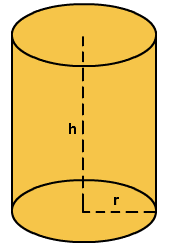The image above represents a cylinder.
To compute the volume of a cylinder requires two essential parameters which are the radius and height of the cylinder.

The formula for computing the volume of a cylinder is:

V = πr2h

Where:
V = Volume of a cylinder
r = radius of the cylinder
h = height of the cylinder

Let’s solve an example
Find the volume of a cylinder with a radius of 3 cm and a height of 5 cm.

This implies that:
r = radius of the cylinder = 3
h = height of the cylinder = 5

V = πr2h
V = 3.142 x 32 x 5
V = 141.39

Therefore, the volume of the cylinder is 141.39 cm3.

Calculating the Height of a cylinder when Volume and Radius is Given

The formula is h = V / πr2

Where;
V = Volume of a cylinder
r =  radius of the cylinder
h = height of the cylinder

Let’s solve an example:
Find the height of a cylinder with a volume of 300 cm3 and a radius of 3 cm

This implies that;
V = Volume of the cylinder = 300 cm3
r  = radius of the cylinder = 3 cm

h = V / πr2
h = 300 / 3.142(3)2
h = 300 / 28.278
h = 10.61
Therefore, the height of the cylinder is 10.61 cm.

Calculating the Radius of a cylinder when Volume and Height is Given

The formula is r = √(V / πh)

Where;
V = Volume of a cylinder
r =  radius of the cylinder
h = height of the cylinder

Let’s solve an example:
Find the radius of a cylinder with a volume of 200 cm3 and a height of 5 cm

This implies that;
V = Volume of the cylinder = 200 cm3
h  = height of the cylinder = 5 cm

r = √(V / πh)
r = √(200 / 3.142(5))
r = √(200 / 15.71)
r = √12.73
r = 3.57

Therefore, the radius of the cylinder is 3.57 cm.

## How to Calculate the Area, Radius and Height of a Cylinder | Nickzom Calculator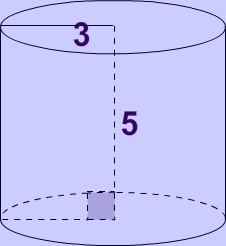The image above represents a cylinder.
To compute the area of a cylinder requires two essential parameters which are the radius and height of the cylinder.

The formula for computing the area of a cylinder is:

A = 2πrh + 2πr2

Where:
A = Area of a cylinder
r = radius of the cylinder
h = height of the cylinder

Let’s solve an example
Find the area of a cylinder with a radius of 3 cm and a height of 5 cm.

This implies that:
r = radius of the cylinder = 3
h = height of the cylinder = 5

A = 2πrh + 2πr2
A = 2(3.142) x 3 x 5 + 2(3.142) x 32
A = 150.82

Therefore, the area of the cylinder is 150.82 cm2.

Calculating the Height of a cylinder when Area and Radius is Given

The formula is h =  A/ 2πr – r

Where;
A = Area of a cylinder
r =  radius of the cylinder
h = height of the cylinder

Let’s solve an example:
Find the height of a cylinder with an area of 600 cm² and a radius of 5 cm

This implies that;
A = Area of the cylinder = 600 cm²
r  = radius of the cylinder = 5 cm

h = A / 2πr – r
h = 600 / 2(3.142)(5) – 5
h = 600 / 31.42 – 5
h = 19.10 – 5
h = 14.10

Therefore, the height of the cylinder is 14.10 cm.

## How to Calculate and Solve for the Perimeter or Circumference, Radius and Diameter of a Circle | The Calculator Encyclopedia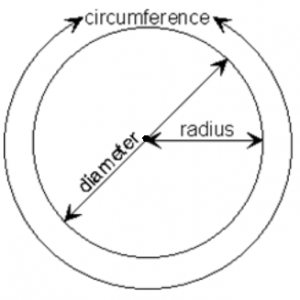The image above is a circle.

To compute the perimeter or circumference of a circle, one essential parameter is needed and this parameter is the radius of a circle (r). You can also use the diameter of a circle to compute the area of a circle (d).

The formula for calculating the perimeter or circumference of a circle is:

P = 2πr

Where:

P = Perimeter or Circumference of a circle
r = Radius of a circle

Let’s solve an example:
Find the perimeter or circumference of a circle where the radius of a circle is 8 cm.

This implies that;
r = Radius of a circle = 8 cm

P = 2πr
P = 2 x 3.142 x 8
P = 50.265

Therefore, the perimeter or circumference of a circle is 50.265 cm.

Calculating the Area of a Circle using the Diameter of a Circle.

The formula is P = πd

Where:

P = Perimeter or Circumference of a circle
d = Diameter of a circle

Let’s solve an example:
Find the perimeter or circumference of a circle where the diameter of a circle is 10 cm.

This implies that;
d = Diameter of a circle = 10 cm

P = πd
P = 3.142 x 10
P = 31.42

Therefore, the perimeter or circumference of a circle with diameter is 31.42 cm.

How to Calculate Radius of a Circle when Perimeter or Circumference of the Circle is Given

r = P /

where;

r = Radius of a circle
P = Perimeter or Circumference of a circle

Let’s solve an example:
Find the radius of a circle where the perimeter or circumference of the circle is 16 cm.

This implies that;
P = Perimeter or Circumference of the circle = 16 cm

r = P /
r = 16 / 6.284
r = 2.55

Therefore, the radius of the circle is 2.55 cm.

How to Calculate Diameter of a Circle when Perimeter or Circumference of the Circle is Given

d = P / π

where;

d = Diameter of a circle
P = Perimeter or Circumference of a circle

Let’s solve an example;
Find the diameter of a circle where the perimeter or circumference of the circle is 20 cm

This implies that;
P = Perimeter or Circumference of the circle = 20 cm

d = P / π
d = 20 / π
d = 6.365

Therefore, the diameter of the circle is 6.365 cm.

## How to Calculate and Solve for the Area and Length of a Parallelogram | The Calculator EncyclopediaThe image above represents a parallelogram.
To compute the area of a parallelogram requires two essential parameters which are the base and height of the parallelogram.

The formula for computing the area of a parallelogram is:

A = b x h

Where:
A = Area of a parallelogram
b = base of the parallelogram
h = height of the parallelogram

Let’s solve an example
Find the area of a parallelogram with a base of 12 cm and a height of 4 cm.

This implies that:
b = base of the parallelogram = 12
h = height of the parallelogram = 4

A = b x h
A = 12 x 4
A = 48

Therefore, the area of the parallelogram is 48 cm2.

Calculating the base of a parallelogram when Area and Height is Given

The formula is b = A / h

Where;
A = Area of a parallelogram
b = base of the parallelogram
h = height of the parallelogram

Let’s solve an example:
Find the base of a parallelogram with an area of 40 cm² and a height of 8 cm

This implies that;
A = Area of the parallelogram = 40 cm²
h = height of the parallelogram = 8 cm

b = A / h
b = 40 / 8
b = 5

Therefore, the base of the parallelogram is 5 cm.

Calculating the height of a parallelogram when Area and Base is Given

The formula is h = A / b

Where;
A = Area of a parallelogram
b = base of the parallelogram
h = height of the parallelogram

Let’s solve an example:
Find the height of a parallelogram with an area of 60 cm² and a base of 6 cm

This implies that;
A = Area of the parallelogram = 60 cm²
b = base of the parallelogram = 6 cm

h = A / b
h = 60 / 6
h = 10

Therefore, the height of the parallelogram is 10 cm.

## How to Calculate and Solve for the Area, Base and Height of a Triangle | The Calculator Encyclopedia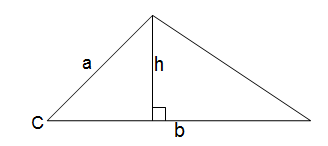The image above is a triangle.
To compute the area of a triangle two essential parameters are required which are the base of the triangle and the height of the triangle.

The formula for calculating the area of a triangle is:

A = 0.5bh

Where:

A = Area of a Triangle
b = Base of a Triangle
h = Height of a Triangle

Let’s solve an example:
Given that the base of a triangle is 12 cm and the height of the triangle is 4 cm. Find the area of the triangle.

This implies that:
b = Base of the Triangle = 12 cm
h = Height of the Triangle = 4 cm

A = 0.5bh
A = 0.5(12)(4)
A = 0.5(48)
A = 24

Therefore, the area of the triangle is 24 cm2

How to Calculate the Base of a Triangle when the Area and Height of the Triangle is Given

The formula is:

b = 2A / h

Where:

b = Base of the Triangle
A = Area of the Triangle
h = Height of the Triangle

Let’s take an example:
Find the base of a triangle when the height and area of the triangle is 12 cm and 40 cm2 respectively.

This implies that:
A = Area of the Triangle = 40 cm2
h = Height of the Triangle = 12 cm

b = 2(40) / 12
b = 80 / 12
b = 6.667

Therefore, the base of the triangle is 6.667 cm.

How to Calculate the Height of a Triangle when the Area and Base of the Triangle is Given

The formula is:

h = 2A / b

Where:

## How to Calculate and Solve for the Volume and Length of a Cube | The Calculator Encyclopedia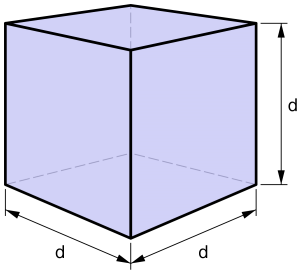The image above is a cube.

To compute the volume of a cube, one essential parameter is needed and this parameter is the length of the cube (l).

The formula for calculating the volume of a cube is;

V = l3

Where;

V = Volume of a cube
l = Length of a cube

Let’s solve an example:
Find the volume of a cube where the length of a cube is 4 cm.

This implies that;
l = length of the cube = 4 cm.

V = l3
V = 43
V = 64 cm3

Therefore, the volume of the cube is 64 cm3

Calculating the Length of a cube using the Volume of the cube.

The formula is l = 3√V

Where;

V = Volume of a cube
l = length of a cube

Let’s solve an example:
Find the length of a cube where the volume of the cube is 120 cm3

This implies that;
V = Volume of the cube = 120 cm3

l = 3√V
l = 3√120
l = 4.93

Therefore, the length of the cube is 4.93 cm.

## How to Calculate and Solve for the Perimeter, Length and Width of a Rectangle | The Calculator EncyclopediaThe image above is a rectangle with a length of 8 cm and a width of 5 cm.
To compute the perimeter of a rectangle requires two parameters which are the length of the rectangle and the width of the rectangle.

The formula for calculating the perimeter of a rectangle is:

P = 2 (l + w)

Where:

P = Perimeter of a Rectangle
l = Length of a Rectangle
w = Width of a Rectangle

Let’s solve an example:
Find the perimeter of a rectangle where the length of the rectangle is 8 cm and the width of the rectangle is 5 cm.

This implies that:
l = Length of the Rectangle = 8 cm
w = Width of the Rectangle = 5 cm

P = 2 (l + w)
P = 2 (8 + 5)
P = 2 (13)
P = 26

Therefore, the perimeter of the rectangle is 26 cm.

How to Calculate the Length of a Rectangle when the Perimeter of the Rectangle and the Width of the Rectangle is Given

l = P / 2 – w

Where:
l = Length of the Rectangle
P = Perimeter of the Rectangle
w = Width of the Rectangle

## How to Calculate and Solve for the Area, Length and Height of a Trapezium | The Calculator Encyclopedia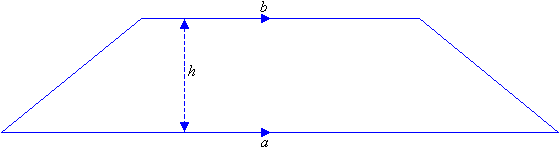The image above is a Trapezium

To compute the area of a trapezium, three essential parameter is needed and they are the length of the top side (a), length of the bottom side (b) and the height of the trapezium (h).

The formula for calculating the area of a trapezium is;

A = 0.5[a + b]h

Where;
A = Area of a trapezium
a = length of the top side of the trapezium
b = length of the bottom side of the trapezium
h = height of the trapezium

Let’s solve an example;
Find the area of a trapezium where length of top side (a) is 7 cm, length of bottom side (b) is 12 cm and height of trapezium (h) is 10 cm.

This implies that;
a = Length of top side of the trapezium = 7 cm
b = Length of bottom side of the trapezium = 12 cm
h = Height of the trapezium = 10 cm

A = 0.5[a + b]h
A = 0.5[7 + 12]10
A = 0.510
A = 95

Therefore, the area of a trapezium is 95 cm2

How to Calculate the Height of a Trapezium when the Area, Length of top side and Length of bottom side of the Trapezium is given.

The formula is h = A / 0.5(a + b)

Where;
A = Area of the trapezium
a = Length of the top side of the trapezium
b = Length of the bottom side of the trapezium

Let’s solve for an example;
Given that the length of top side (a) is 10 cm, length of bottom side (b) is 14 cm and the area of the trapezium is 20 cm2 Find the height of the trapezium?

This implies that;

A = Area of the trapezium = 20 cm2
a = Length of top side of the trapezium = 10 cm
b = Length of bottom side of the trapezium = 14 cm

h = A / 0.5(a + b)
h = 20 / 0.5(10 + 14)
h = 20 / 0.5(24)
h = 20 / 12
h = 1.667

Therefore, the height of the trapezium is 1.667 cm.

## How to Calculate and Solve for the Perimeter and Length of a Square | The Calculator Encyclopedia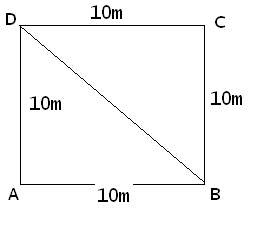The image above represents a square.
To compute the perimeter of a square requires one essential parameter which is the length of one side of a square.

The formula for computing the perimeter of a square is:

P = 4 x l

Where:
P = Perimeter of a Square
l = Length of one side of a Square

Let’s solve an example
Find the perimeter of a square which has a length of 10 m.

This implies that:
l = length of one side of a square = 10

P = 4 x l
P = 4 x 10
P = 40

Therefore, the perimeter of the square is 40 m.

Calculating the Length of a Square when Perimeter is Given

The formula is l = p / 4

Where;
P = Perimeter of a square
l = Length of a square

Let’s solve an example:
Find the length of a square where the perimeter of a square is 100 m.

This implies that;
P = Perimeter of a square = 100 m

l = p / 4
l = 100 / 4
l = 25

Therefore, the length of the square is 25 m.

## How to Calculate and Solve for the Area, Width and Length of a Rectangle | The Calculator Encyclopedia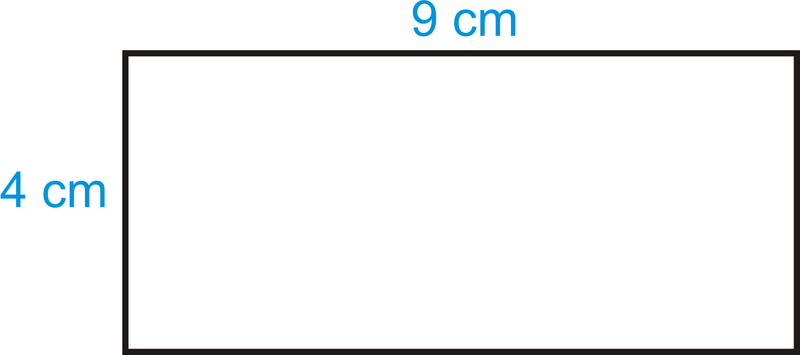The image above is a rectangle with a length of 9 cm and a width of 4 cm.
To compute the area of a rectangle requires two essential parameters which are the length of the rectangle and the width of the rectangle.

The formula for calculating the area of a rectangle is:

A = lw

Where:

A = Area of a Rectangle
l = Length of a Rectangle
w = Width of a Rectangle

Let’s solve an example
Find the area of a rectangle where the length of the rectangle is 9 cm and the width of the rectangle is 4 cm.

This implies that:
l = Length of the Rectangle = 9 cm
w = Width of the Rectangle = 4 cm

A = lw
A = (9)(4)
A = 36

Therefore, the area of the rectangle is 36 cm2.

How to Calculate the Length of a Rectangle when the Area and Width of the Rectangle is Given

The formula for calculating the length of the rectangle is:

l = A / w

Where:

l = Length of the Rectangle
A = Area of the Rectangle
w = Width of the Rectangle

Let’s solve an example:
Find the length of a rectangle where the area of the rectangle is 45 cm2 and the width of the rectangle is 9 cm.

This implies that:
A = Area of the Rectangle = 45 cm2
w = Width of the Rectangle = 9 cm

l = A / w
l = 45 / 9
l = 5

Therefore, the length of the rectangle is 5 cm.

How to Calculate the Width of a Rectangle when the Area and Length of the Rectangle is Given

The formula for calculating the width of the rectangle is:

w = A / l

Where:

w = Width of the Rectangle
A = Area of the Rectangle
l = Length of the Rectangle